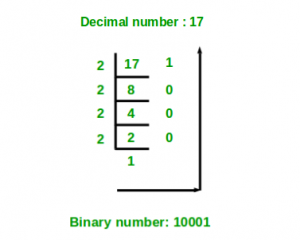# Decimal to binary number using recursion

Given a decimal number as input, we need to write a program to convert the given decimal number into equivalent binary number.

Examples :

```Input : 7
Output :111

Input :10
Output :1010```

## Recommended: Please try your approach on {IDE} first, before moving on to the solution.

We have discussed one iterative solution in below post.
Program for Decimal to Binary Conversion

Below is Recursive solution

```findBinary(decimal)
if (decimal == 0)
binary = 0
else
binary = decimal % 2 + 10 * (findBinary(decimal / 2)```

.Step by step process for better understanding of how the algorithm works
Let decimal number be 10.
Step 1-> 10 % 2 which is equal-too 0 + 10 * ( 10/2 ) % 2

Step 2-> 5 % 2 which is equal-too 1 + 10 * ( 5 / 2) % 2

Step 3-> 2 % 2 which is equal-too 0 + 10 * ( 2 / 2 ) % 2

Step 4-> 1 % 2 which is equal-too 1 + 10 * ( 1 / 2 ) % 2

## C++

 `// C++ program for decimal to binary ` `// conversion using recursion ` `#include ` `using` `namespace` `std; ` ` `  `// Decimal to binary conversion ` `// using recursion ` `int` `find(``int` `decimal_number) ` `{ ` `    ``if` `(decimal_number == 0)  ` `        ``return` `0;  ` `    ``else` `        ``return` `(decimal_number % 2 + 10 *  ` `                ``find(decimal_number / 2)); ` `} ` ` `  `// Driver code  ` `int` `main() ` `{ ` `    ``int` `decimal_number = 10; ` `    ``cout << find(decimal_number); ` `    ``return` `0; ` `} ` `// This code is contributed by shivanisinghss2110 `

## C

 `// C/C++ program for decimal to binary ` `// conversion using recursion ` `#include ` ` `  `// Decimal to binary conversion ` `// using recursion ` `int` `find(``int` `decimal_number) ` `{ ` `    ``if` `(decimal_number == 0)  ` `        ``return` `0;  ` `    ``else` `        ``return` `(decimal_number % 2 + 10 *  ` `                ``find(decimal_number / 2)); ` `} ` ` `  `// Driver code  ` `int` `main() ` `{ ` `    ``int` `decimal_number = 10; ` `    ``printf``(``"%d"``, find(decimal_number)); ` `    ``return` `0; ` `} `

## Java

 `// Java program for decimal to binary ` `// conversion using recursion ` `import` `java.io.*; ` ` `  `class` `GFG  ` `{ ` `     `  `    ``// Decimal to binary conversion  ` `    ``// using recursion ` `    ``static` `int` `find(``int` `decimal_number) ` `    ``{ ` `        ``if` `(decimal_number == ``0``)  ` `            ``return` `0``;  ` `             `  `        ``else` `         `  `        ``return` `(decimal_number % ``2` `+ ``10` `*  ` `                ``find(decimal_number / ``2``)); ` `    ``} ` `     `  `// Driver Code ` `public` `static` `void` `main(String args[]) ` `{ ` `    ``int` `decimal_number = ``10``; ` `    ``System.out.println(find(decimal_number)); ` `} ` ` `  `} ` ` `  `// This code is contributed by Nikita Tiwari `

## Python3

 `# Python3 code for decimal to binary ` `# conversion using recursion ` ` `  `# Decimal to binary conversion  ` `# using recursion ` `def` `find( decimal_number ): ` `    ``if` `decimal_number ``=``=` `0``: ` `        ``return` `0` `    ``else``: ` `        ``return` `(decimal_number ``%` `2` `+` `10` `*`  `                ``find(``int``(decimal_number ``/``/` `2``))) ` ` `  `# Driver Code ` `decimal_number ``=` `10` `print``(find(decimal_number)) ` ` `  `# This code is contributed ` `# by "Sharad_Bhardwaj" `

## C#

 `// C# program for decimal to binary ` `// conversion using recursion ` `using` `System; ` ` `  `class` `GFG  ` `{ ` `     `  `    ``// Decimal to binary conversion  ` `    ``// using recursion ` `    ``static` `int` `find(``int` `decimal_number) ` `    ``{ ` `        ``if` `(decimal_number == 0)  ` `            ``return` `0;  ` `             `  `        ``else` `         `  `        ``return` `(decimal_number % 2 + 10 *  ` `                ``find(decimal_number / 2)); ` `    ``} ` `     `  `    ``// Driver Code ` `    ``public` `static` `void` `Main() ` `    ``{ ` `         `  `        ``int` `decimal_number = 10; ` `         `  `        ``Console.WriteLine(find(decimal_number)); ` `    ``} ` `} ` ` `  `// This code is contributed by vt_m `

## PHP

 ` `

Output :

```1010
```

Attention reader! Don’t stop learning now. Get hold of all the important DSA concepts with the DSA Self Paced Course at a student-friendly price and become industry ready.

My Personal Notes arrow_drop_upCheck out this Author's contributed articles.

If you like GeeksforGeeks and would like to contribute, you can also write an article using contribute.geeksforgeeks.org or mail your article to contribute@geeksforgeeks.org. See your article appearing on the GeeksforGeeks main page and help other Geeks.

Please Improve this article if you find anything incorrect by clicking on the "Improve Article" button below.